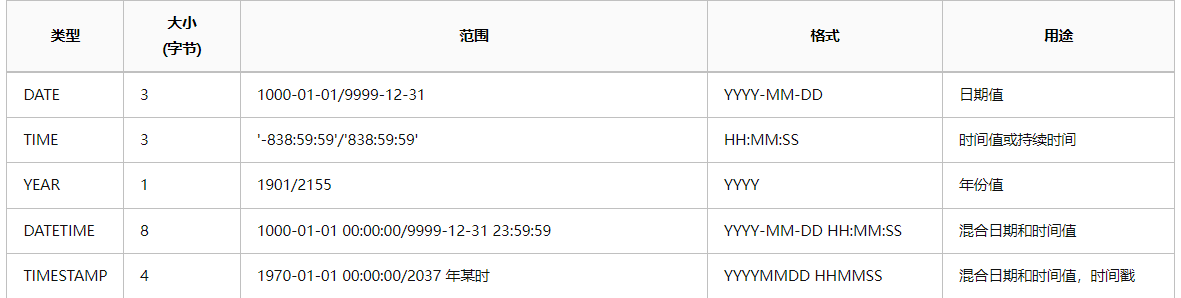墨滴2021/11/20  阅读：22  主题：默认主题

# mysql的日期函数的处理

mysql 有 5 种表示时间值的日期和时间类型

mysql 有 5 种表示时间值的日期和时间类型，分别为、DATE，TIME，YEAR，DATETIME，TIMESTAMP

``MySQL 数据类型               含义 date                     只存储年月日，'2018-07-12'time                     只存储时分秒，'09:51:02'datetime 存储年月日 时分秒，'2018-07-12 09:51:02'timestamp            存储年月日 时分秒，'2018-07-12 09:51:02'   注：这个类型的字段时间会随着其他字段值的修改，自动刷新year                     只存储年，'2018'``主要的还是对mysql的日期函数的使用先说mysql获取当前时间的几种方法

``  SELECT CURDATE() //获取是年月日 SELECT NOW()//获取是年月日  时分秒 SELECT CURRENT_DATE() //获取是年月日 SELECT SYSDATE()//获取是年月日  时分秒``

``SELECT * FROM kq_clock  WHERE  create_time=CURDATE()SELECT * FROM kq_clock WHERE   attendance_date=NOW()SELECT * FROM kq_clock WHERE   attendance_date=SYSDATE()``

``select TIMESTAMP('2010-03-03 16:41:16'); //2010-03-03 16:41:16select DATE('2010-03-03 16:41:16'); //2010-03-03select YEAR('2010-03-03 16:41:16'); //2021select MONTH('2010-03-03 16:41:16'); //3select DAY('2010-03-03 16:41:16'); //3select TIME('2010-03-03 16:41:16'); //16:41:16SELECT WEEK(NOW())//47  获取本年第几周SELECT MONTHNAME(NOW())//获取日期月份的英文SELECT DAYNAME(NOW())//返回是英文名称``

``SELECT * FROM clock_log log INNER JOIN clock_time  time on  log.rules_id=time.rule_idWHERE  DAYNAME(NOW()) in ( SELECT DISTINCT SUBSTRING_INDEX(SUBSTRING_INDEX(weekdays,',',b.help_topic_id + 1),',',-1) as  gFROM  clock_time  INNER JOIN mysql.help_topic b ON b.help_topic_id < (LENGTH(clock_time.weekdays) - LENGTH(REPLACE(clock_time.weekdays, ',', '')) + 1)  WHERE  clock_time.rule_id=time.rule_id)``

``SELECT DATE_ADD('1999-01-01', INTERVAL 1 DAY)  //加一天SELECT DATE_ADD(NOW(), INTERVAL 1 HOUR);//加一个小时SELECT DATE_ADD(NOW(), INTERVAL 1 MONTH);//加一个月``

``SELECT * FROM order  WHERE create_time>date_add(                        '2021-11-02 ',INTERVAL SUBSTRING_INDEX("09:00:00", ':', 1) HOUR)``

SUBSTRING_INDEX截取字符串以:这个考研用于time时分秒的字段 加一个小时

``SELECT DATE_SUB('1999-01-01', INTERVAL 1 DAY)  //减一天SELECT DATE_SUB(NOW(), INTERVAL 1 HOUR);//减一个小时SELECT DATE_SUB(NOW(), INTERVAL 1 MONTH);//减一个月``

``SELECT * FROM order  WHERE create_time>date_sub(                        '2021-11-02 ',INTERVAL SUBSTRING_INDEX("09:00:00", ':', 1) HOUR)``

``SELECT * FROM order  WHERE create_time>date_sub(date_add(                        '2021-11-02',INTERVAL SUBSTRING_INDEX("09:00:00", ':', 1) HOUR)                        ,INTERVAL SUBSTRING_INDEX("09:00:00", ':', 1) HOUR)``

date_format()函数

//需求1 查询这个月 8：00到 9：00的订单的数据呢

``SELECT * FROM  `order` WHERE  date_format(create_time,'%H:%i')>='09:00'  and  date_format(create_time,'%H:%i')<='19:00' and date_format(create_time,'%Y-%m-%d')=DATE_FORMAT(NOW(),'%Y-%m-%d')  GROUP BY log.employee_id``

``SELECT log.employee_id FROM kq_clock_log log INNER JOIN kq_clock_time  time on  log.rules_id=time.rule_idWHERE   DATE_FORMAT(MIN(log.create_time),'%H:%i:%S')<=time.leave_time  and   DATE_FORMAT(min(log.create_time),'%H:%i:%S')>=time.work_timeand   DATE_FORMAT((log.create_time),'%H:%i:%S')= DATE_FORMAT(NOW(),'%Y-%m-%d')  GROUP BY  log.employee_id``

time.leave_time 规定的上班时间 time格式

work_time 规定的下班时间 time格式 但是 我要判断这个人最早的数据是不是在这个时间端中 上面的sql不能使用

`` DATE_FORMAT(MIN(log.create_time),'%H:%i:%S')<=time.leave_time  and   DATE_FORMAT(min(log.create_time),'%H:%i:%S')>=time.work_time``

``SELECT log.employee_id FROM clock_log log INNER JOIN clock_time  time on  log.rules_id=time.rule_idWHERE   DATE_FORMAT((log.create_time),'%H:%i:%S')<=time.leave_time  and  log.employee_id not  in ( SELECT log.employee_id FROM clock_log log INNER JOIN clock_time  time on  log.rules_id=time.rule_id where  DATE_FORMAT((log.create_time),'%H:%i:%S')<=time.work_time and  DATE_FORMAT((log.create_time),'%H:%i:%S')= DATE_FORMAT(NOW(),'%Y-%m-%d')  GROUP BY log.employee_id)and   DATE_FORMAT((log.create_time),'%H:%i:%S')>=time.work_time and   DATE_FORMAT((log.create_time),'%H:%i:%S')= DATE_FORMAT(NOW(),'%Y-%m-%d')  GROUP BY  log.employee_id``

`` DATE_FORMAT(MIN(log.create_time),'%H:%i:%S')<=time.leave_time  and   DATE_FORMAT(min(log.create_time),'%H:%i:%S')>=time.work_time``2021/11/20  阅读：22  主题：默认主题